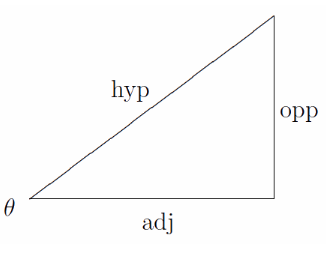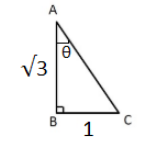# Cot-Tan formula

The law of cot or Tangent which is also called as a cot-tangent formula or cot-tangent rule is the ratio of the cot of the angle to the cos of the angle in tangent formula

Tan Theta = Opposite Side / Adjacent Side

Cot Theta = Adjacent Side/ Opposite Side

## Cot – Tan x formula

The Tan θ is the ratio of the Opposite side to the Adjacent, where (θ) is one of the acute angles.## Solved Examples

Out of other 6 trigonometric formulas, let’s have a look at the practice question of tan theta formula.

Example 1: Show that (cosec A – sin A)(sec A – cos A) = 1/ (tan A + cot A)

Solution:
LHS = (cosec A – sin A)(sec A – cos A)
= [(1/sin A) – sin A][(1/cos A) – cos A] [(1 – sin2A)/sin A][(1 – cos2A)/cos A] = (cos2A/sin A)(sin2A/cos A)
= sin2A cos2A/sin A cos A
= sin A cos A

RHS = 1/(tan A + cot A)
= 1/[tan A + (1/tan A)] = tan A/ (tan2A + 1)
= tan A/sec2A
= tan A cos2A
= (sin A/cos A) cos2A
= sin A cos A
Therefore, LHS = RHS
(cosec A – sin A)(sec A – cos A) = 1/ (tan A + cot A)

Example 2: If √3 tan θ = 1, then find the value of cot2θ + sin2θ – cos2θ.

Solution:
Given,
√3 tan θ = 1
tan θ = 1/√3
cot θ = √3AC = √(3 + 1) = √4 = 2
sin θ = ½
cos θ = √3/2
cot2θ + sin2θ – cos2θ = (√3)2 + (½)2 – (√3/2)2
= 3 + ¼ – ¾
= (12 + 1 – 3)/4
= 5/2

To practice more Trigonometric questions and understand the trigonometric concepts better, register on BYJU’S.